Courses

# Test: Aromaticity

## 20 Questions MCQ Test Chemistry for JEE | Test: Aromaticity

Description
This mock test of Test: Aromaticity for JEE helps you for every JEE entrance exam. This contains 20 Multiple Choice Questions for JEE Test: Aromaticity (mcq) to study with solutions a complete question bank. The solved questions answers in this Test: Aromaticity quiz give you a good mix of easy questions and tough questions. JEE students definitely take this Test: Aromaticity exercise for a better result in the exam. You can find other Test: Aromaticity extra questions, long questions & short questions for JEE on EduRev as well by searching above.
QUESTION: 1

### Direction (Q. Nos. 1 - 8) This section contains 8 multiple choice questions. Each question has four choices (a), (b), (c) and (d), out of which ONLY ONE option is correct. Q. Arrange the following compounds in increasing order of polarity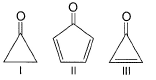Solution:

In case 1, the bond is broken in oxygen’s favor and it will attain its octet. Also, carbon becomes sp2 hybridized, so there is a chance of polarity.
In case 2, if the bond is broken in favor of oxygen, then the ring will become anti-aromatic which is highly unstable and the bond won’t be broken in that way. If the bond is broken in favor of carbon in the ring, then although the ring becomes aromatic but oxygen will bear +ve charge which is very unstable. So, there is no chance to break the bond.
In case 3, if the double bond is broken in favor of oxygen, then oxygen will acquire negative charge and the ring will become aromatic. So, it is a highly favorable case of double bond breaking.
Therefore, the order of polarity: - III>I>II

QUESTION: 2

### In principle, what is true regarding benzene and 1, 3, 5-cyclohexatriene?

Solution:

The correct answer is Option D.
In 1,3,5-cyclohexatriene, there are three C = C having a bond length 134 pm and three C - C having a bond length 154 pm. In benzene, all the six C - C bonds have the same bond length 139 pm.

QUESTION: 3

### Enthalpy of hydrogenation of cyclohexene is -119 kJ/mol and that of benzene is -208 kJ/mol. Based on these information, resonance energy of benzene can be calculated to be

Solution:

The correct answer is Option C.
ΔHhyd (cyclohexene) = - 119 kJ/mol (1C = C)
ΔHexp (benzene) = -3 × 119 = -357 (3C = C)
ΔHcal (benzene) = -208 KJ/mol
∴ resonance energy = ΔHexp - ΔHcal
= -357 + 208
= -149 kJ/mol

QUESTION: 4

Select the species which is not aromatic.

Solution: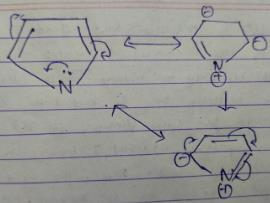Here, we can see that Nitrogen gave its lone pair to make the system aromatic. The same case happens with option b and d. But with option c, there is no lone pair with Boron. So option c is correct answer

QUESTION: 5

Consider the following bromides :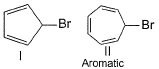Solution:

In the compound I, Br will dispatch as Br+ so that a -ve charge appears on carbon which will give us 6π electrons. So ring will become aromatic.(4n+2 π electron is needed for aromaticity)
In compound II, Br will dispatch as Br-. So that carbon has +ve charge and all the double bond will circulate in the ring. This will maintain 4n+2 π electron and the molecule will remain as aromatic.

QUESTION: 6

Which is not true regarding 1, 3, 5, 7-cyclooctatetraene?

Solution:

The correct answer is Option D.

It is anti aromatic system with 8 s hence d option is wrong.

QUESTION: 7

The following compound , when treated with excess of AgBF4 gives a red precipitate leaving a highly conducting filtrate, due to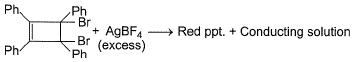Solution:

The correct answer is Option B.
An aromatic dication is formed, the no of  s become 6 thus it obeys 4n+2 rule hence it is aromatic and highly stable.

QUESTION: 8

When potassium metal is added to 1, 3, 5, 7-cyclooctatetraene, a highly conducting salt is formed without evolution of H2 gas because

Solution:
QUESTION: 9

Direction (Q. Nos. 9 - 12) This section contains 4 multiple choice questions. Each question has four choices (a), (b), (c) and (d), out of which ONE or MORE THAN ONE are correct.

When considering electrophilic aromatic substitution reactions the halides are described as

Solution:
QUESTION: 10

What makes the following compound aromatic?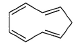Solution:

To make the given compound aromatic, we will remove an H+ from sp3 hybridized carbon atom to get 10 cyclic electrons (i.e. electrons move around the periphery of the ring).

*Multiple options can be correct
QUESTION: 11

What is true about the compound calicene?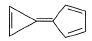Solution:
*Multiple options can be correct
QUESTION: 12

Which of the following species is/are anti-aromatic?

Solution:

The correct options are A & D

Anti Aromatic compounds are those compounds which satisfy the rules of planarity and fully conjugation of the pi electrons inside the ring but fail to satisfy Huckel's rule of 4n+2 pi electrons.
Anti-aromatic compound contains 4nπ electrons.
Here the options A and D are anti-aromatic.

QUESTION: 13

Direction (Q. Nos. 13 - 17) This section is based on Statement i and Statement II. Select the correct answer from the codes given below.

Q.

Statement I : Benzene has very high stability than a general triene.

Statement II : Benzene is a completely conjugated system.

Solution:
QUESTION: 14

Statement I : Pyrene, although aromatic, decolourise brown colour of bromine water.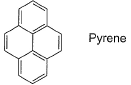Statement II : It has one pi-bond extra which is not the part of aromatic system.

Solution:
QUESTION: 15

Statement I : The follow compound is optically active.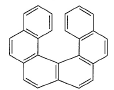Statement II : It has a chiral carbon.

Solution:

The correct answer is option C

Statement 1 is correct because Plane Of Symmetry and Centre Of Symmetry are absent in the compound .
Statement 2 is clearly incorrect as there is No 'Sp3 ' Hybridized Carbon atom .

QUESTION: 16

Statement I : Furan is an aromatic system, has resonance energy comparable to that of benzene.

Statement II : Furan decolourises the brown colour of bromine water solution.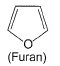Solution:

Furan has resonance energy comparable to benzene. But the double bonds are involved in aromaticity, so it won’t decolourise Bromine water.

QUESTION: 17

Arrange the halogens F2, Cl2, Br2, I2 in order of their increasing reactivity with alkanes.

Solution:

The correct answer is Option A.
Since reactivity decreases down the group as the electronegativity of the halogen decreases down the group. Thus, rate of reaction of alkanes with halogens is
I2 < Br2 < Cl2 <F2

*Answer can only contain numeric values
QUESTION: 18

Direction (Q. Nos. 18 - 20) This section contains 3 questions. When worked out will result in an integer from 0 to 9 (both inclusive)
Cyclobutene when refluxed in presence of potassium metal, evolve hydrogen gas and an aromatic system is formed. How many pi-electrons are involved in the above formed aromatic system?

Solution:
QUESTION: 19

When two or more different substituents are attached with a benzene ring the number 1 position in the ring is given to a high priority group. Which one of the following groups has highest - priority?

Solution:
*Answer can only contain numeric values
QUESTION: 20

The compound below has four phenyl rings, but very less stable due to an opposing factor of stability. Therefore, this compound absorb bromine in dark.
How many bromine molecules, when added to this molecule, would make it stable and prevent further bromine addition?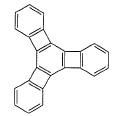[IIT JEE 2005]

Solution:

• Test